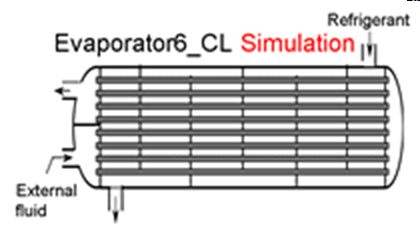Contents - index

Evaporator6_CLEvaporator6_CL determines the outlet state of a refrigerant evaporator of specified size.  Thermal energy is transferred from an external fluid, which may be a liquid such as water or a brine.  It is necessary to provide the heat transfer coefficients on the external fluid side of the heat exchanger and for the saturated and superheated sections on the refrigerant side.  These heat transfer coefficients can be determined using the functions in the Heat Transfer Library, as illustrated in example for Evaporator5_CL.

This library file can be used in the design stage of the evaporator by inputing the design inlet conditions to determine the required evaporator size.  Use Evaporator6_CL to determine the refrigerant and external fluid outlet states for given inlet conditions and evaporator area.

Inputs:

R\$:   name of the refrigerant

m_dot_R:  refrigerant flow rate (kg/s, lbm/hr)

h_R_in:  specific enthalpy of entering refrigerant (J/kg, kJ/kg, Btu/lbm)

P_R:  refrigerant pressure (Pa, kPa, bar, MPa, psia, atm)

Config\$: a string constant or string variable that must be either 'counterflow' or 'parallelflow'

m_dot_fluid:  mass flow rate of the external fluid  (kg/s, lbm/hr)

T_fluid_in:  external fluid inlet temperature  (C, K, F, R)

cp_fluid:  specific heat of external fluid (J/kg-K, kJ/kg-K, Btu/lbm-R)

h_fluid:  effective outside (external fluid) heat transfer coefficient per unit refrigerant tube area (W/m^2-K, Btu/hr-ft^2-R)

A_fluid\A_R:  ratio of effective heat transfer area on the external fluid side to the heat transfer area for the refrigerant*

h_R_sat:  average heat transfer coefficient for refrigerant in the saturated regime  (W/m^2-K, Btu/hr-ft^2-R)

h_R_sh:  average heat transfer coefficient for refrigerant in the superheated regime  (W/m^2-K, Btu/hr-ft^2-R)

A_R:  required heat transfer area for the refrigerant (m^2, ft^2)

Outputs:

Q_dot:  overall heat transfer rate to evaporator (W, kW, Btu/hr)

h_R_out refrigerant outlet specific enthalpy (J/kg, kJ/kg, Btu/lbm)

T_fluid_out:  outlet temperature of the external fluid  (C, K, F, R)

*A_fluid\A_R is the ratio of the product of the overall fin efficiency and total (finned and unfinned) area on the external fluid side to the total surface area inside the refrigerant flow passages.  For unfinned surfaces, A_fluid\A_R is the ratio of the outer surface to inner surface areas.

Notes:

In actual operation, the heat exchanger type may shell-in-tube, plate or other type.  However, Config\$ may only be set to 'Parallelflow' or 'Counterflow'.  These two options provide upper and lower bounds on the required heat transer area.

Example:

\$UnitSystem SI C kPa kJ mass

R\$='R134a' "refrigerant"

m_dot_R=0.08 [kg/s] "refrigerant flow rate"

T_cond_out=38 [C]  "exit temperature of refrigerant from condenser"

P_cond=1 [MPa]*convert(MPa,kPa)  "condenser pressure"

h_cond_out=enthalpy(R\$,T=T_cond_out,P=P_cond)  "specific enthalpy of refrigerant exiting condenser"

P_R=200 [kPa]  "evaporator pressure"

T_R_sat=temperature(R\$,P=P_R,x=0)  "evaporator saturation temperature"

h_R_in=h_cond_out  "specific enthalpy of refrigerant entering evaporator"

x_R_in=quality(R\$,h=h_R_in,P=P_R)  "inlet quality"

Fluid\$='EG' "ethylene glycol-water is the external fluid"

Conc=30 [%] "concentration of the ethylene glycol - water solution"

T_fluid_in=5 [C]  "inlet temperature of external fluid"

Config\$='counterflow'  {parallelflow} "heat exchanger configuration;  counterflow and parallelflow bound the problem"

m_dot_fluid=0.5 [kg/s] "mass flow rate of external fluid"

cp_fluid=cp(EG,T=T_fluid_in,C=Conc) "specific heat of external fluid"

DELTAT_sh=5 [DELTAK]  "degrees of superheat"

h_R_sh=205 [W/m^2-K]  "convection coefficient for superheated R134a."

h_R_sat=540 [W/m^2-K]  "convection coefficient for saturated R134a - see calculation of h_cond below"

h_fluid=475 [W/m^2-K]  "convection coefficient for external fluid  "

A_fluid\A_R=1.186 "ratio of area on the external fluid side to that on the refrigerant side"

A_R=3.60 [m^2] "evaporator surface area"

Call evaporator6_cl(R\$, m_dot_R, h_R_in, P_R, Config\$, m_dot_fluid, T_fluid_in, cp_fluid, h_fluid, A_fluid\A_R,  h_R_sat, h_R_sh, A_R :  Q_dot, h_R_out, T_fluid_out)

{Solution

A_fluid\A_R=1.186 [-]

A_R=3.6 [m^2]

Conc=30 [%]

Config\$='counterflow'

cp_fluid=3.673 [kJ/kg-K]

DELTAT_sh=5 [DELTAK]

Fluid\$='EG'

h_cond_out=105.3 [kJ/kg]

h_fluid=475 [W/m^2-K]

h_R_in=105.3 [kJ/kg]

h_R_out=248.6 [kJ/kg]

h_R_sat=540 [W/m^2-K]

h_R_sh=205 [W/m^2-K]

m_dot_fluid=0.5 [kg/s]

m_dot_R=0.08 [kg/s]

P_cond=1000 [kPa]

P_R=200 [kPa]

Q_dot=11.47 [kW]

R\$='R134A'

T_cond_out=38 [C]

T_fluid_in=5 [C]

T_fluid_out=-1.244 [C]

T_R_sat=-10.09 [C]

x_R_in=0.3245

}